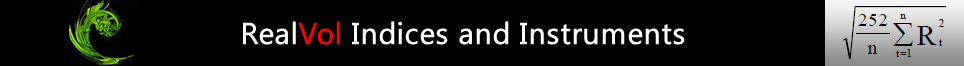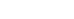# RealVol Real-Time Formula(Realized Volatility Formulas)

The RealVol real-time formula is used to create the RealVol real-time index (VOL).  This index provides an indication of the 1-month daily realized volatility index (1RVOL) throughout the trading day.

### Design of RealVol Real-Time Formula

All of the design elements described for the RealVol Daily Formula are the same for the RealVol Real-Time Formula. To convert from a daily to a real-time value, one needs to start with the RealVol Daily Formula, then incorporate the current underlying price and a weighting scheme. Doing so provides continuous updates throughout the trading day and delivers to traders a useful, real-time indication of the up-to-the-moment 21-day daily realized volatility. At VolX, it was decided to convert only the 1RVOL Index to a real-time index (VOL). Essentially, VOL measures a constant 21-day realized volatility even while we are within the new, most recent, day (“Today”).For instance, if we are 80% through the current day (n+1), we will use the most up-to-the-moment URP to calculate the partial (80%) day’s return (n+1) from yesterday’s URP (n). Then we consider the very first day in the calculation period and weight that whole day’s return by 20% (100% - 80% = 20%). In this manner, we still have the weight of 21-day realized volatility at any moment in time even though there are actually 22 returns — 20% weight on day 1, 80% weight on day 22, and full weights for days 2 through 21 (for a total weight of 21 days of returns).

Note: While the partial return of the current day is self-weighting, and therefore requires no additional coefficient, the self-weighted portion of the current day is nonetheless required to be calculated so as to apply the proper remaining weight to the full day 1 return.  In order to calculate the weight of the current day, the current time each day is measured to the closest second. Since there are 86,400 seconds in a day, the current time and the number of seconds in a day are used in the RealVol Real-Time Formula to calculate the daily weight to be applied to day 1.

When the time of day equals the close of today (n+1), the weight of the return of day n+1 is now 100%, while the weight of the return of day 1 is 0%. Thus, with its weight of zero, the return of the original day 1 drops out of the calculation. The original day 2 now becomes the new day 1 and all other days get renumbered as well. The RealVol Real-Time Formula at this very instant in time (the close at 4:00 PM ET in our example) simplifies to the RealVol Daily Formula. The instant after the market closes, we begin anew, with the returns renumbered, such that there are again only 21 returns, with the new trading day having a weighted return as day 22.

Note that the new “day” begins immediately after the close of the market on a particular day (ignoring holidays and weekends), not necessarily at the end of the day (i.e., midnight).

### Formula 3Where:

86,400 = number of seconds in a day
n+1 = today
s = number of seconds up to the current moment in time of the current day (n+1) beginning from the time of the most recent market close (day n), excluding intervening weekend days and holidays
R1 = return for first day (day 1) of the period (from close day zero to close day 1)
Rn+1 = partial return (the return using the current underlying price and the Underlying Reference Price of the prior day).  Note:  For clarification, the italic “R” denotes partial return; all other returns are full-day returns.

Also see RealVol Daily Formula.